# Arrhenius Equation & Activation Energy Notes | Study Physical Chemistry - Chemistry

## Chemistry: Arrhenius Equation & Activation Energy Notes | Study Physical Chemistry - Chemistry

The document Arrhenius Equation & Activation Energy Notes | Study Physical Chemistry - Chemistry is a part of the Chemistry Course Physical Chemistry.
All you need of Chemistry at this link: Chemistry

Arrhenius Equation (Expression)

The following empirical relationship between temperature (T), rate constant (k) and activation energy (Ea) is known as the Arrhenius expression:

K = Ae-Ea / RT

A is constant known as the frequency factor or Arrhenius pre-exponential factor.
A & Ea is temperature independent.
The unit of A is always equal to the unit of rate constant (k) k = Ae-Ea / RT ..................(1)
taking natural log of this equation, we get ln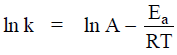…(2)

or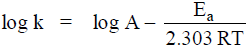…(3)

Problem. Prove that on increasing the activation energy, the rate constant will be decreasing and on increasing the temperature, the rate constant will be increasing.
Sol:

k = Ae-Ea / RT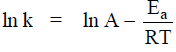when Ea increase then the value of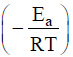ncreases and the value of ln k i.e. k will be decreases.
i.e.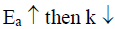When T increase then the value of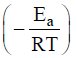decreases and the value of ln k i.e. k will be increase.
i.e.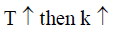The graph of ln k vs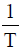is given below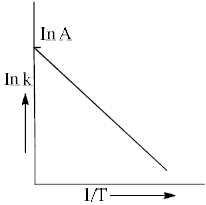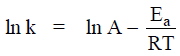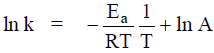y = –m. x + C

Problem.  Using the given equation find the value of A & Ea.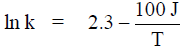Sol. We know that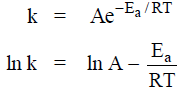i.e.           ln A = 2.3
&        A = e2.3 = 9.974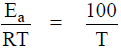E= 100 × R = 100 × 8.314

Ea = 831.4 J mol–1

Problem.  When temperature is increased then t1/2 of reaction will be

(a) Remains constant               (b) Increased

(c) Decreased                           (d) First increase and then decrease

Sol. We know that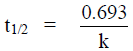&               k = Ae-Ea / RT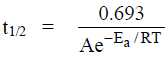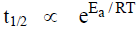i.e. on increasing T, eEa / RT decrease then we can say that on increasing temperature (T), the t 1/2 of the reaction will decrease. i.e.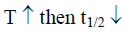i.e. The correct answer is (C).

Variation of rate constant with temperature

We know that k = Ae-Ea / RT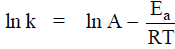If k1 and k2 be the value of rate constant at temperature Tand T2, we can derive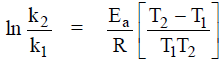or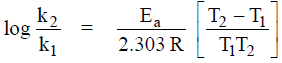Temperature Coefficient.

The ratio of rate constant of a reaction at two different temperature differing by 10 degree is know as temperature coefficient.
i.e. Temperature coefficient =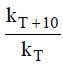Standard Temperature coefficient =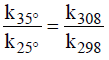= 2 to 3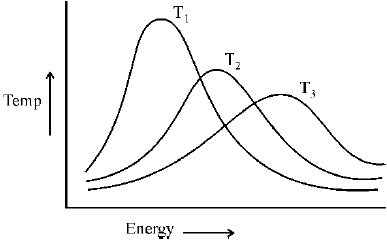Problem.  In the reaction mechanism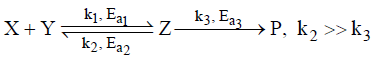Find the overall rate constant (koverall) and Activation energy Ea (overall).
Sol.
From the above reaction, the ate of format ion of product is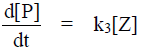......(1)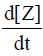= k1[X][Y] – k2[Z] – k3[Z]
= k1[X][Y] – (k2 + k3)[Z]
0 = k1[X][Y] – k2[Z]                                                             [∵ k2 >> k3]

SSA on intermediate.
then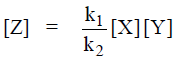then we find,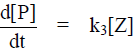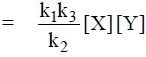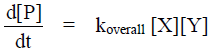i.e.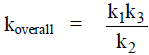…(2)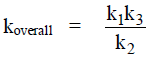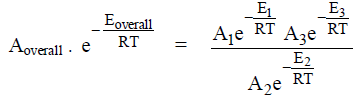i.e.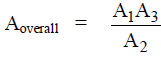and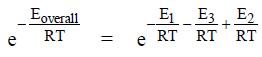i.e.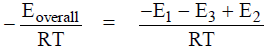Eoverall = E1 + E3 – E2

Problem.  What is the energy of activation of the reaction if it rate doubles when temperature is raised .290 to 300 K.
Sol.
We know that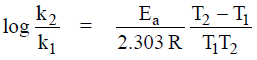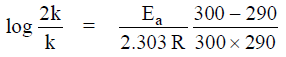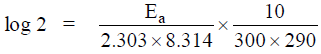Ea = 50145.617 J
Ea = 50.145 kJ

Problem. A plot of log k versus 1/T gave a straight line of which the slope was found to be –1.2 × 104 K. What is the activation energy of the reaction.
Sol.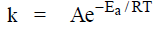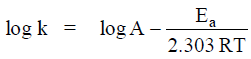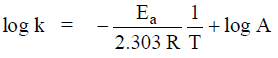y = m x + C

where             m = slope of line
then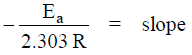E= –2.303 R (slope) = –2.303 × 8.314 × (–1.2 × 104 K)
= 1.0 × 105 J mol–1

Fast Reaction.

Fast reactions are studies by following methods
(1)  Stopped-Flow technique: For reaction that occur on timescales as short at 1 ms (10–3 s)
(2) Flash photolysis technique: Reaction that can be triggered by light are studied using flash photolysis.
(3)  Perturbation-relaxation methods: A chemical system initially at equilibrium is perturbed such that the system is not longer at equilibrium. By following the relaxation of the system back toward equilibrium, the rate constant for the reaction can be determined.
The temperature perturbation or T-jump are most important type of perturbation.

Problem. Using the T-jump method find out the relaxation time (ζ) of the following reaction,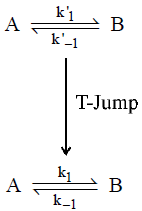Sol.  Let a be the total concentration of (A + B) and x the concentration of B at any instant. Then rate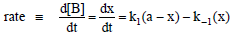If xe is the equilibrium concentration, then
Δx = x - xe or x = Δx + xe
Since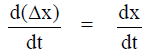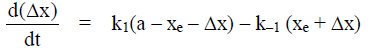at equilibrium,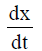= 0 and x = xe. Hence

k1(a – xe) = k–1 xe

then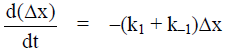= -krΔx
where              k= k1 + k-1
= relaxat ion rate constant
then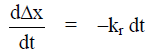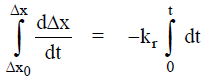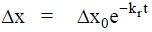Then reciprocal of kr i.e. kr-1 is called relaxat ion time.
It is represented by ζ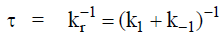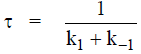Problem.  Find the relaxation time for the following reaction.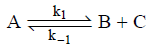Sol. Let a be the total concentration and x the concentration of B which is equal to the concentration of C. Then, the rate law is given by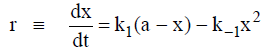Now             Δx = x – xe

xe = equilibrium concentration of x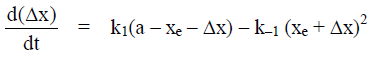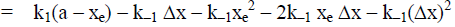at equilibrium,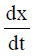= 0, hence

we get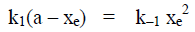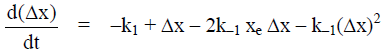Δx is very small than (Δx)2 is neglected,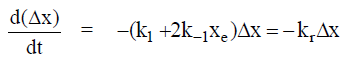where               kr = k1 + 2k–1 xe
is the relaxation rate constant.
and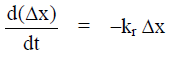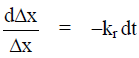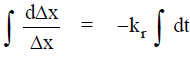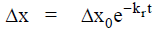The relaxat ion time ζ in this case is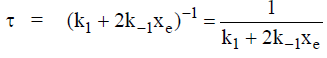Problem.  The relaxation time for the fast reaction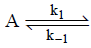is 10 µs and the equilibrium constant is 1.0 × 10–3. Calculate the rate constant for the forward and the reverse reactions.
Sol.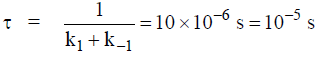K = equilibrium constant = 1.0 × 10–3 =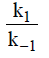∴ k1 = 1.0 × 10–3 k–1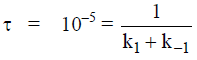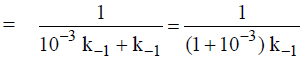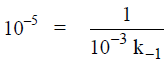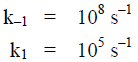The document Arrhenius Equation & Activation Energy Notes | Study Physical Chemistry - Chemistry is a part of the Chemistry Course Physical Chemistry.
All you need of Chemistry at this link: ChemistryUse Code STAYHOME200 and get INR 200 additional OFF

## Physical Chemistry

83 videos|106 docs|31 tests

Track your progress, build streaks, highlight & save important lessons and more!

,

,

,

,

,

,

,

,

,

,

,

,

,

,

,

,

,

,

,

,

,

;